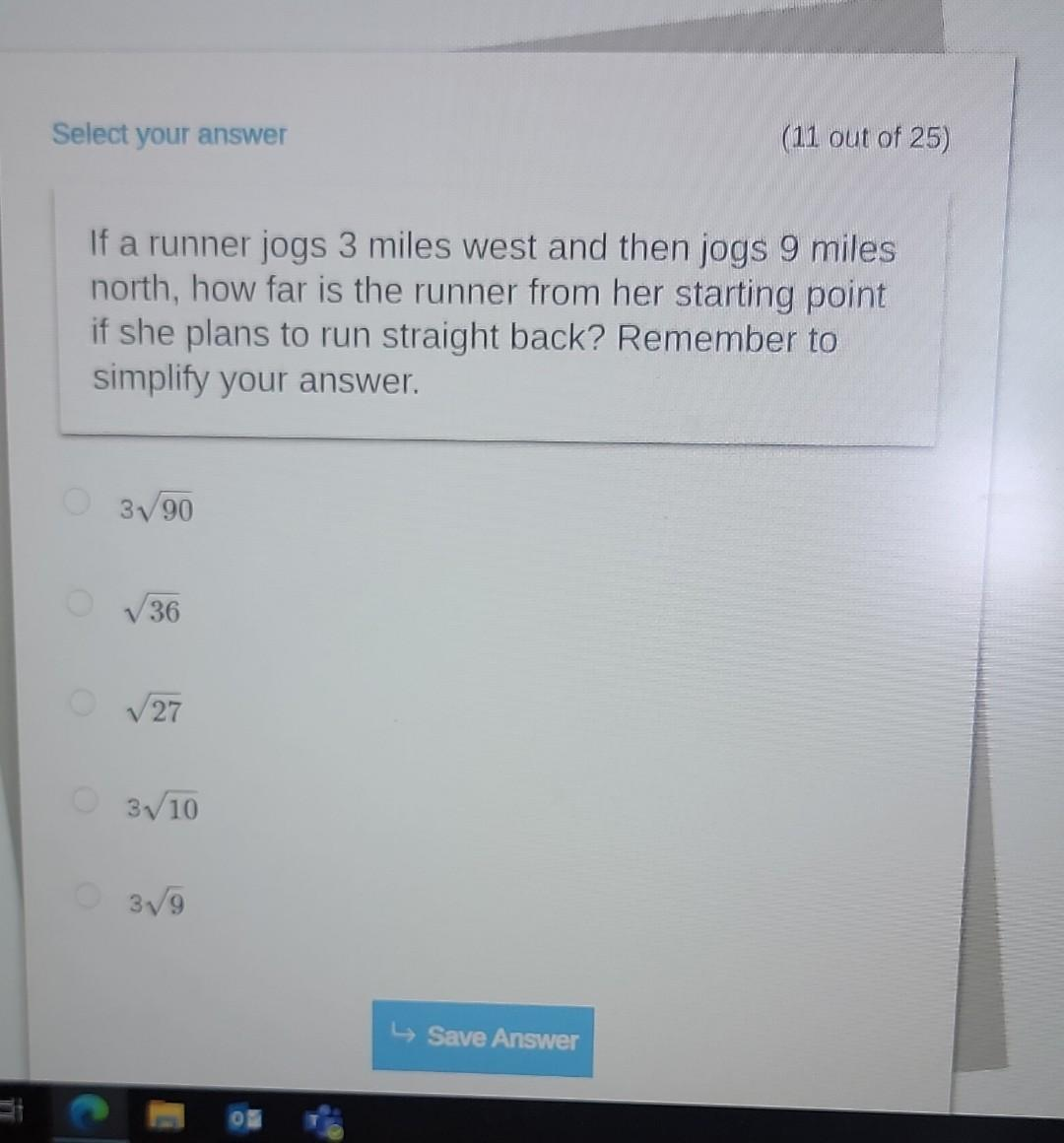Home / Expert Answers / Algebra / nbsp-if-a-runner-jogs-3-miles-west-and-then-jogs-9-miles-north-how-far-is-the-runner-from-her-pa216

# (Solved):   If a runner jogs 3 miles west and then jogs 9 miles north, how far is the runner from her ...If a runner jogs 3 miles west and then jogs 9 miles north, how far is the runner from her starting point if she plans to run straight back? Remember to simplify your answer. $$3 \sqrt{90}$$ $$\sqrt{36}$$ $$\sqrt{27}$$ $$3 \sqrt{10}$$ $$3 \sqrt{9}$$

We have an Answer from Expert